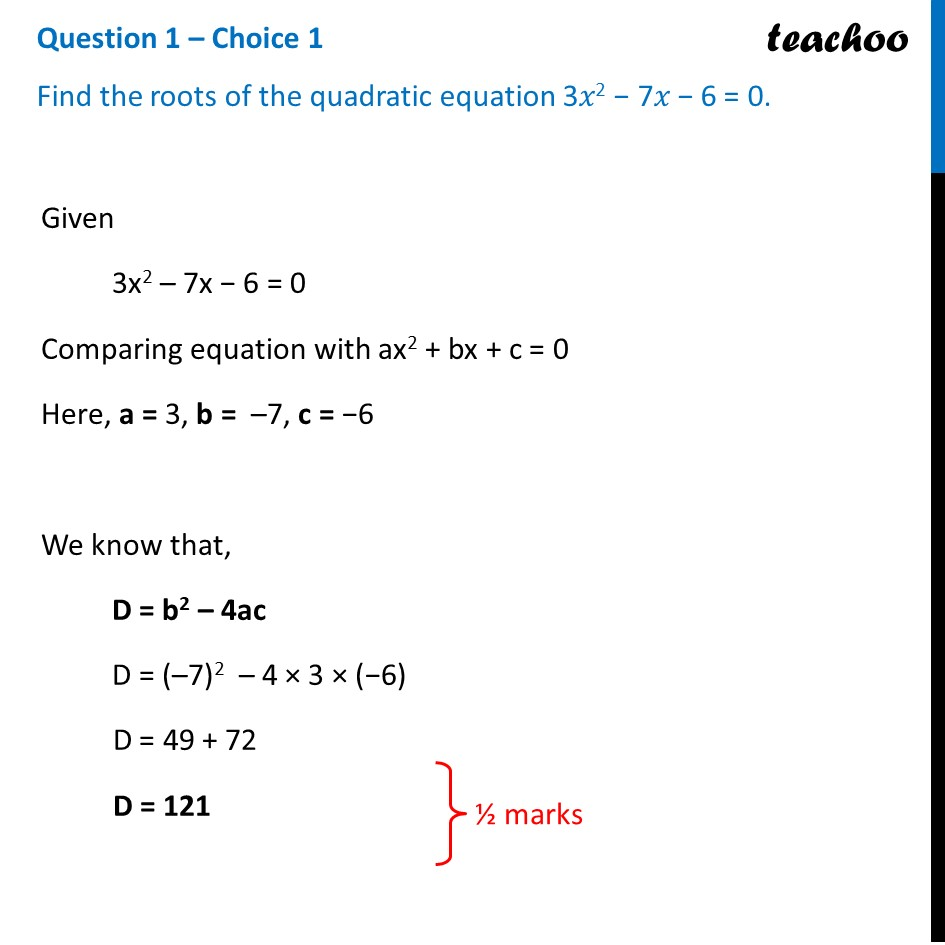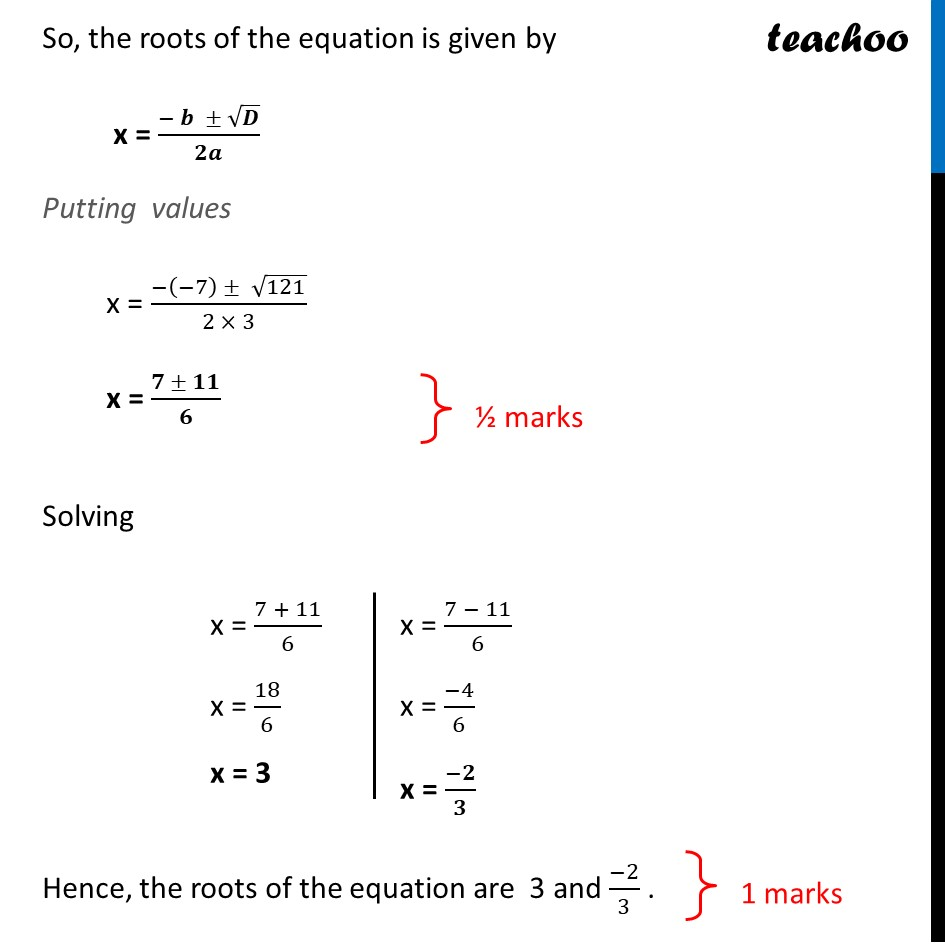CBSE Class 10 Sample Paper for 2022 Boards - Maths Basic [Term 2]

Class 10
Solutions of Sample Papers for Class 10 Boards

## Find the roots of the quadratic equation 3x 2 - 7x - 6 = 0

This question is similar to Example 13 (i) Chapter 4 Class 10 - Quadratic EquationsGet live Maths 1-on-1 Classs - Class 6 to 12

### Transcript

Question 1 – Choice 1 Find the roots of the quadratic equation 3𝑥2 − 7𝑥 − 6 = 0. Given 3x2 – 7x − 6 = 0 Comparing equation with ax2 + bx + c = 0 Here, a = 3, b = –7, c = −6 We know that, D = b2 – 4ac D = (–7)2 – 4 × 3 × (−6) D = 49 + 72 D = 121 So, the roots of the equation is given by x = (− 𝒃 ± √𝑫)/𝟐𝒂 Putting values x = (−(−7) ± √121)/(2 × 3) x = (𝟕 ± 𝟏𝟏)/𝟔 Solving Hence, the roots of the equation are 3 and (−2)/3 .